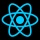Home
IT Knowledge
Inspiration
Languages
EN

# Python - remove set items

0 points
Created by:Admin Dirask Community
1810

In this article, we would like to show you how to remove set items in Python.

Quick solution:

``my_set.remove('item')``

or

``my_set.discard('item')``

Note:

The difference between `remove()` and `discard()` method is that when the item to remove doesn't exist, the `discard()` method won't throw an error.

## 1. Practical example using `remove()` method

In this example, we use `remove()` method to remove `B` item from the set.

``````my_set = {'A', 'B', 'C'}

my_set.remove('B')

print(my_set)  # {'C', 'A'}``````

Output:

``{'C', 'A'}``

## 2. Using `discard()` method

In this example, we use `discard()` method to remove `B` item from the set.

``````my_set = {'A', 'B', 'C'}

print(my_set)  # {'C', 'A'}``````

Output:

``{'A', 'C'}``

## 3. Clear the set

In this example, we use `clear()` method to empty the set.

``````my_set = {'A', 'B', 'C'}

my_set.clear()

print(my_set)  # set()``````

Output:

``set()``